# How to Delete an Element at a Particular Index in a given Array

## How to Delete an Element at a Particular Index in a given Array in C:

In this article, I am going to discuss Deletion in an Array i.e. how to delete an element at a particular index in a given array using both C and C++ Language with Examples. Please read our previous article, where we discussed How to Display Append and Insert Elements in an Array.

##### Understanding the Concept i.e. Deletion in an Array:

Here, we will learn how to delete or remove a particular element from an array using both C and C++ Programming Languages. An array is a collection of elements of the same data type stored in a contiguous memory location. In C programming language, an array is derived data that stores primitive data type values like int, char, float, etc. To delete a specific element from an array, we must define the position from which the array’s element should be removed. The deletion of the element does not affect the size of an array. Furthermore, we should also check whether the deletion is possible or not in an array.

For example, suppose an array contains 8 elements, arr[] = {10, 25, 14, 8, 12, 15, 5); and the user want to delete element 8. So, first, the user must define the position of the 8th element, which is the 4th, and then check whether the deletion is possible or not. The position of the particular element should not be more than the total elements of an array. Here, we have 7 elements in an array, and the user wants to delete the 8th position element, which is impossible.

##### Steps to Delete an Element at a Particular Index in a given Array

Let’s create an array of size 10 and set the length of the array to 0 as there is no element inside our array.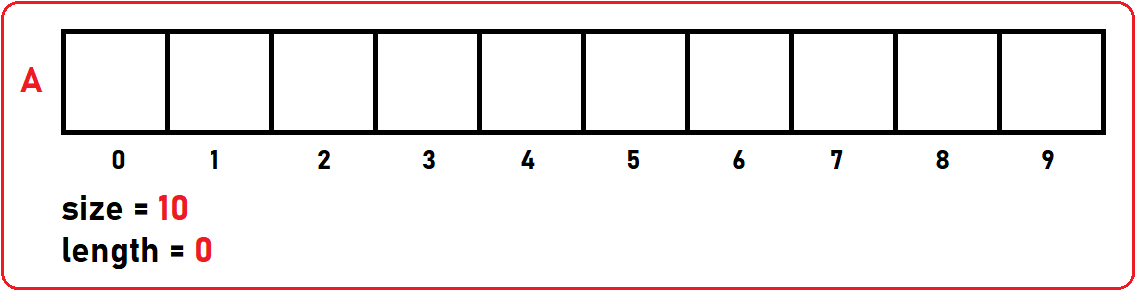Now, we insert some elements in the above array. Here, we insert 6 elements, so the length of the array will also 6.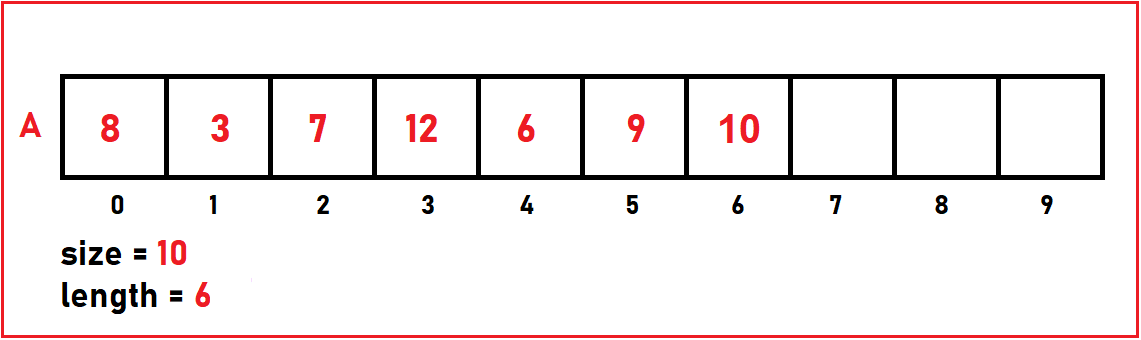Now, I want to delete an element at a given index from the above array. Let’s take the example of Delete (4). In the above array, 6 is stored at the 4th index. So, we have to remove six. To perform the deletion, we have to follow the below steps:

1. First, store the element in another variable that you want to delete from the array. Int x = arr ;
2. Second, when we delete an element from an array, those elements which are present after the element which we have deleted, we have to right shift these elements by 1, so that there will no empty space inside our array.

In the above array, as we have already stored that element in another variable, now we can proceed to shift. In the below image, we are shifting 9 from index 5 to 4.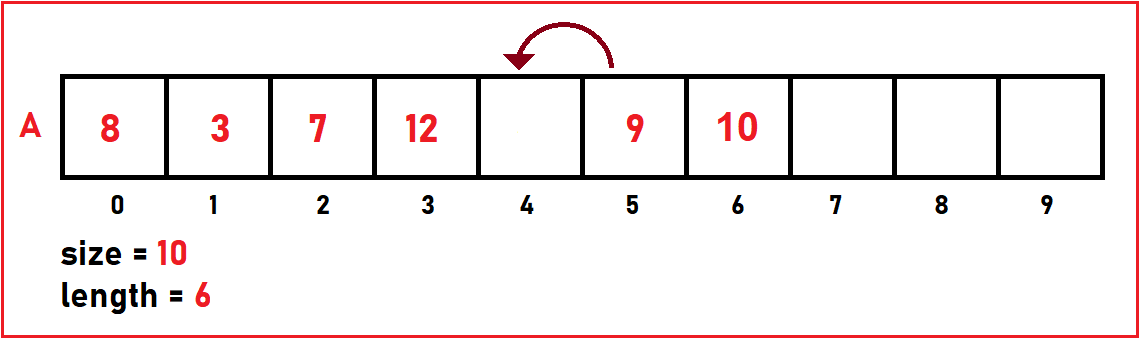Then, we are shifting 10 from index 6 to 5.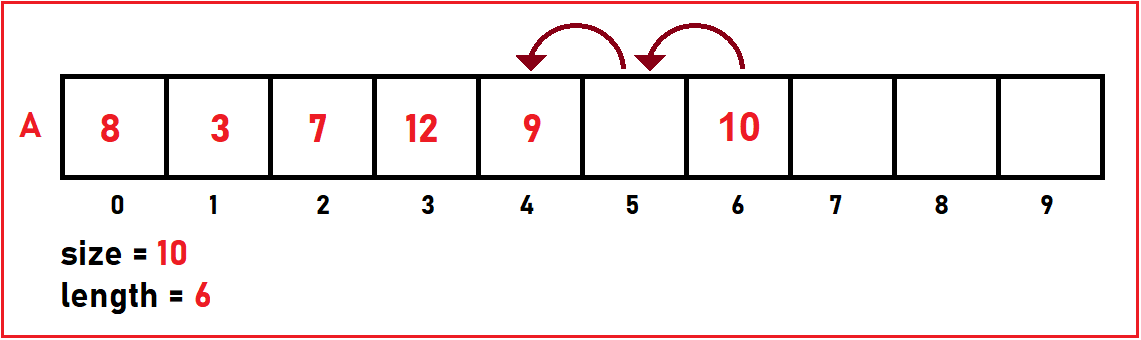As we have shifted both the elements, we have to decrease the length by 1 because here we deleted one element from the given array.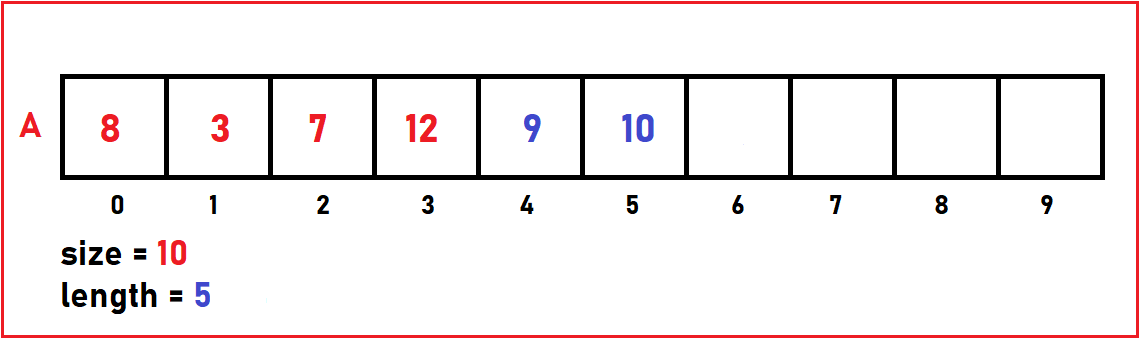Now let’s see how we can do this in C language.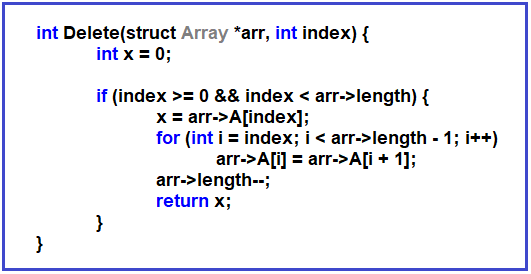In deletion operation, we know,

1. The minimum shifting is 1 i.e. when we want to delete the last 2nd element
2. The maximum shifting Is n i.e. when we want to delete the first element of the array.
3. The minimum time is constant and the maximum time is n to perform the deletion.
##### Example to Delete an Element at a Particular Index in a given Array using C Language:
```#include <stdio.h>
#include <stdlib.h>

struct Array {
int* A;
int size;
int length;
};

int Delete(struct Array* arr, int index) {
int x = 0;

if (index >= 0 && index < arr->length) {
x = arr->A[index];
for (int i = index; i < arr->length - 1; i++)
arr->A[i] = arr->A[i + 1];
arr->length--;
return x;
}
}

int main() {
struct Array arr;

printf("Enter Size of an Array: ");
scanf("%d", &arr.size);

arr.A = (int*)malloc(sizeof(int) * arr.size);
arr.length = 0;

printf("Enter Number of Elements: ");
scanf("%d", &arr.length);

printf("Enter All Elements: \n");
for (int i = 0; i < arr.length; i++) {
scanf("%d", &arr.A[i]);
}

printf("\nDeleted Element is : %d\n", Delete(&arr, 4));

getchar();
}
```
###### Output: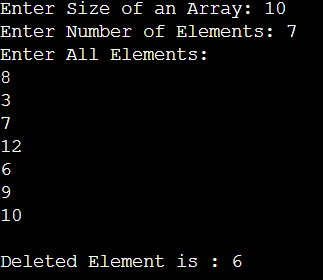##### Example to Delete an Element at a Particular Index in a given Array using C++ Language:
```#include <iostream>
#include <conio.h>
using namespace std;

struct Array {
int* A;
int size;
int length;
};

int Delete(struct Array* arr, int index) {
int x = 0;

if (index >= 0 && index < arr->length) {
x = arr->A[index];
for (int i = index; i < arr->length - 1; i++)
arr->A[i] = arr->A[i + 1];
arr->length--;
return x;
}
}

int main() {
struct Array arr;

cout << "Enter Size of an Array: ";
cin >> arr.size;

arr.A = new int[arr.size];
arr.length = 0;

cout << "Enter Number of Elements: ";
cin >> arr.length;

cout << "Enter All Elements: \n";
for (int i = 0; i < arr.length; i++) {
cin >> arr.A[i];
}

cout << "\nDeleted Element is : " << Delete(&arr, 4);

getchar();
}
```
###### Output: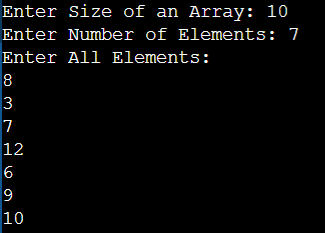In the next article, I am going to discuss Linear Search in Array with Examples. Here, in this article, I try to explain Deletion in an Array i.e. How to DELETE an Element in an Array with Examples in C and C++ Language and I hope you enjoy this Deletion in an Array i.e. How to DELETE an Element in an Array using C and C++ Language with Examples article.